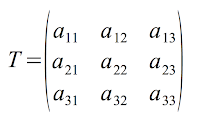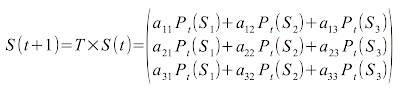## Wednesday, 20 January 2016

### More on Transition matrix and Superposition of states

The example in the previous post was about a very simple 2 states system, now let us consider a bit more complicated system which has 3 states. Things we are going to talk about or calculation we are going to do here for this 3 states system could be easily extended for a system with more than 3 states too. Now look at the following network which can represent any possible network graph of state transitions for a system with 3 states.General state transition network for a 3 states system.

Here you see we have the option of going from any state to any state with a probability. To not get confused we show the probabilities with 'a' instead of 'p' so for any node of the graph the sum of the outgoing arrows should be 1, like the following:

a11 + a21 + a31 = 1
a12 + a22 + a32 = 1
a13 + a23 + a33 = 1

As we talked about the superposition of states in previous considering the following is our transition matrix:Transition matrix for a 3 state system

And the following will give us the next state of the system:New state of the system can be derived by multiplying transition matrix to the current state of the system

Here we remind you again that state of our system at any time is a superposition of possible states, this is why the S(t) itself is a vector of possible states in which any possible state is a vector or matrix itself. It is like in t+1 your system can be in any of these 3 states each with a probability. So the S(t+1) will be something like the following:New state of a system based on the previous state

And again like the previous post we always can go forward and calculate the future states:

S(t)
S(t+1) = T x S(t)
S(t+2) = T x S(t+1) = x ( x S(t) )
.
.
.
S(t+n) = T x S(t+n-1) = x ( T x ( T x ...  x S(t) )

What basically this says is that you can predict the state probabilities of the system in future, regardless of how far the selected future is and how complicate your system is. The more complicated the system the more number of states it has, so the transition matrix grows, and this is why predicting the future of a system like a  single human life or oil price is so much difficult. Because first of all we don't have access to all elements and their values which defines every single state of a human life or oil price or may have some effect on the system and second of all the transition matrix will have millions if not billions of dimensions which practically makes it impossible to get transition calculated with the technologies we have today.

And finally, I provided some tools to draw the network graph for state transitions which also could be useful to draw any network graph. The tools are here "Sleptons Blog Tools" you can use them directly to draw your graphs too like this one "s1,s2,s3,s4,s5,s3,s1,s6,s1,s6" which draws a state sequence.